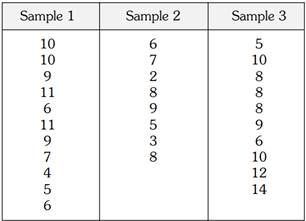### Create an Account

Home / Questions / Consider the following research data State an appropriate null hypothesis What are the val...

# Consider the following research data State an appropriate null hypothesis What are the values of each category mean

Consider the following research data:a. State an appropriate null hypothesis.

b. What are the values of each category mean?

c. What is the value of the grand mean?

d. What is the value of the SSB?

e. What is the value of the SSW?

f. What is the value of the dfB?

g. What is the value of the dfW?

h. What is the value of the MSW?

i. What is the value of the MSB?

j. What is the value of F?

k. Assuming that you were working at the .05 level of significance, what would you conclude?

Apr 25 2020 View more View LessSubscribe To Get Solution